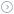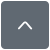### arm条件执行

|

ARM 处理器的一个非常特殊的特征是它的条件执行。我们指的不是基本的如果进位则分支，ARM 使这个逻辑阶段进一步深化为如果进位则 XXX - 这里的 XXX 是任何东西。

JA Jump if Above
JAE Jump if Above or Equal
JB Jump if Below
JBE Jump if Below or Equal
JC Jump if Carry
JCXZ Jump if CX Zero (CX is a register that can be used for loop counts)
JE Jump if Equal
JG Jump if Greater than
JGE Jump if Greater than or Equal
JL Jump if Less than
JLE Jump if Less Than or Equal
JMP JuMP
JNA Jump if Not Above
JNAE Jump if Not Above or Equal
JNB Jump if Not Below
JNBE Jump if Not Below or Equal
JNC Jump if No Carry
JNE Jump if Not Equal
JNG Jump if Not Greater than
JNGE Jump if Not Greater than or Equal
JNL Jump if Not Less than
JNLE Jump if Not Less than or Equal
JNO Jump if Not Overflow
JNP Jump if Not Parity
JNS Jump if Not Sign
JNZ Jump if Not Zero
JO Jump if Overflow
JP Jump if Parity
JPE Jump if Parity Even
JPO Jump if Parity Odd
JS Jump if Sign
JZ Jump if Zero
80386 添加了:
JECXZ Jump if ECX Zero

B 分支
BL 带连接的分支

BEQ Branch if EQual
BNE Branch if Not Equal
BVS Branch if oVerflow Set
BVC Branch if oVerflow Clear
BHI Branch if HIgher
BLS Branch if Lower or the Same
BPL Branch if PLus
BMI Branch if MInus
BCS Branch if Carry Set
BCC Branch if Carry Clear
BGE Branch if Greater than or Equal
BGT Branch if Greater Than
BLE Branch if Less than or Equal
BLT Branch if Less Than
BLEQ Branch with Link if EQual
....
BLLT Branch with Link if Less Than

AL - ALways，缺省条件所以不须指定
NV - NeVer，不是非常有用。你无论如何不要使用这个代码...

EQ : 等于

NE : 不等于

VS : 溢出设置

VC : 溢出清除

HI : 高于(无符号)

LS : 低于或同于(无符号)

PL : 正号

MI : 负号

CS : 进位设置

CC : 进位清除

GE : 大于或等于(有符号)

GT : 大于(有符号)

LE : 小于或等于(有符号)

LT : 小于(有符号)

AL : 总是

NV : 从不

\ 条件执行的一个例子
.loop ; 标记循环开始位置
CMP R0, R10 ; 把 R0 与 R10 相比较
SWINE &40017 ; 不等于: 调用 SWI &40017
ADDNE R0, R0, #1 ; 向 R0 加 1
BNE loop ; 分支到 loop
MOV R10, #0 ; 等于 : 设置 R10 为零
LDMFD R13!, {R0-R12,PC} ; 返回到调用者

SWI 编号就象我写的这样。在 RISC OS 下，它是给 Econet_DoImmediate 的编号。不要字面的接受它，这只是一个例子!

LDMFD R13!, {R0-R12,R14}
MOV PC, R14

『本文转载自网络,版权归原作者所有,如有侵权请联系删除』热门文章 更多footer